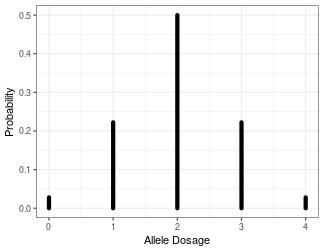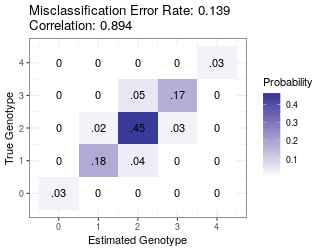# Abstract

We provide some example usage of the oracle calculations available in `updog`. These are particularly useful for read-depth determination. These calculations are described in detail in @gerard2018genotyping.

# Controlling Misclassification Error

Suppose we have a sample of tetraploid individuals derived from an S1 cross (a single generation of selfing). Using domain expertise (either from previous studies or a pilot analysis), we've determined that our sequencing technology will produce relatively clean data. That is, the sequencing error rate will not be too large (say, ~0.001), the bias will be moderate (say, ~0.7 at the most extreme), and the majority of SNPs will have reasonable levels of overdispersion (say, less than 0.01). We want to know how deep we need to sequence.

Using `oracle_mis`, we can see how deep we need to sequence under the worst-case scenario we want to control (sequencing error rate = 0.001, bias = 0.7, overdispersion = 0.01) in order to obtain a misclassification error rate of at most, say, 0.05.

``````bias   <- 0.7
od     <- 0.01
seq    <- 0.001
maxerr <- 0.05
``````

Before we do this, we also need the distribution of the offspring genotypes. We can get this distribution assuming various parental genotypes using the `get_q_array` function. Typically, error rates will be larger when the allele-frequency is closer to 0.5. So we'll start in the worst-case scenario of assuming that the parent has 2 copies of the reference allele.

``````library(updog)
ploidy <- 4
pgeno <- 2
gene_dist <- get_q_array(ploidy = ploidy)[pgeno + 1, pgeno + 1, ]
``````

This is what the genotype distribution for the offspring looks like:

``````library(ggplot2)
distdf <- data.frame(x = 0:ploidy, y = 0, yend = gene_dist)
ggplot(distdf, mapping = aes(x = x, y = y, xend = x, yend = yend)) +
geom_segment(lineend = "round", lwd = 2) +
theme_bw() +
xlab("Allele Dosage") +
ylab("Probability")
``````Now, we are ready to iterate through read-depth's until we reach one with an error rate less than 0.05.

``````err    <- Inf
depth  <- 0
while(err > maxerr) {
depth <- depth + 1
err <- oracle_mis(n = depth,
ploidy = ploidy,
seq = seq,
bias = bias,
od = od,
dist = gene_dist)
}
depth
#>  90
``````

Looks like we need a depth of 90 in order to get a misclassification error rate under 0.05.

Note that `oracle_mis` returns the best misclassification error rate possible under these conditions (`ploidy` = 4, `bias` = 0.7, `seq` = 0.001, `od` = 0.01, and `pgeno` = 2). In your actual analysis, you will have a worse misclassification error rate than that returned by `oracle_mis`. However, if you have a lot of individuals in your sample, then this will act as a reasonable approximation to the error rate. In general though, you should sequence a little deeper than suggested by `oracle_mis`.

# Visualizing the Joint Distribution

Suppose we only have a budget to sequence to a depth of 30. Then what errors can we expect? We can use `oracle_joint` and `oracle_plot` to visualize the errors we can expect.

``````depth <- 30
jd <- oracle_joint(n = depth,
ploidy = ploidy,
seq = seq,
bias = bias,
od = od,
dist = gene_dist)
oracle_plot(jd)
``````Most of the errors will be mistakes between genotypes 2/3 and mistakes between genotypes ½.

Even though the misclassification error rate is pretty high (0.14), the correlation of the oracle estimator with the true genotype is pretty reasonable (0.89). You can obtain this using the `oracle_cor` function.

``````ocorr <- oracle_cor(n = depth,
ploidy = ploidy,
seq = seq,
bias = bias,
od = od,
dist = gene_dist)
ocorr
#>  0.8935101
``````